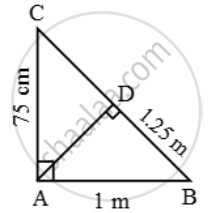# In figure, ∠CAB = 90º and AD ⊥ BC. If AC = 75 cm, AB = 1 m and BD = 1.25 m, find AD. - Mathematics

Sum

In figure, ∠CAB = 90º and AD ⊥ BC. If AC = 75 cm, AB = 1 m and BD = 1.25 m, find AD.

#### Solution

We have,
AB = 1 m = 100 cm, AC = 75 cm and BD = 125 cm

In ∆BAC and ∆BDA, we have

∠BAC = ∠BDA [Each equal to 90º] and, ∠B = ∠B

So, by AA-criterion of similarity, we have

∆BAC ~ ∆BDA\Rightarrow \frac{BA}{BD}=\frac{AC}{AD}

\Rightarrow \frac{100}{125}=\frac{75}{AD}

\Rightarrow AD=\frac{125\times 75}{100}cm=93.75cm

Concept: Similarity
Is there an error in this question or solution?